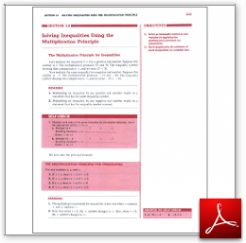Algebra Math Book - Introductory Algebra Thank you for your continual support.

 The power of mathematics rests in the logic of thinking.ID: Sec3-8
Description: Multiplication Principle for Inequalities-Combining the Principles
Price: 1.99

# Algebra Math book - Introductory Algebra - Chapter 3 - Section 8 - Solving Inequalities Using the Multiplication Principle

## Section 3.8 - Objectives

11.  Solve an inequality written in one variable by applying the multiplication principle for inequalities.

12.  Show graphically the solutions for such inequalities on a number line.

Navigate to
Previous Section or Next Chapter
or Chapter 3 Details or Other Chapters

This section of my algebra math book, Introductory Algebra, also includes in the download:

• Cover Sheet